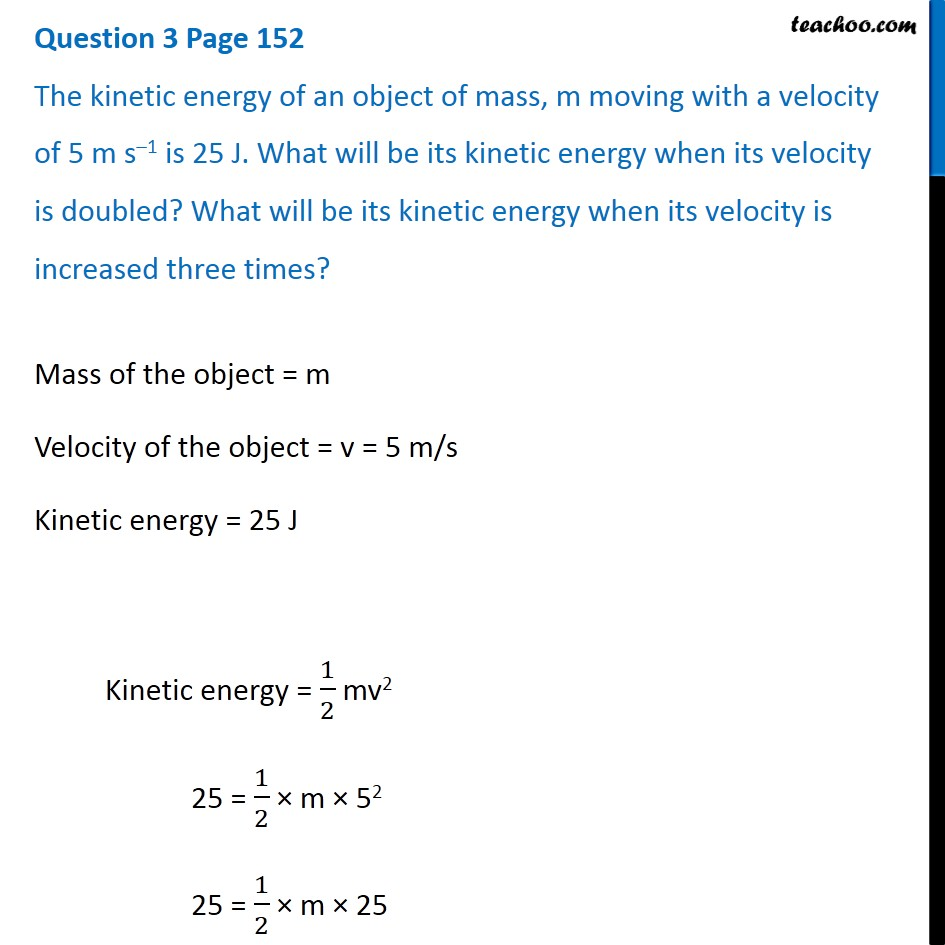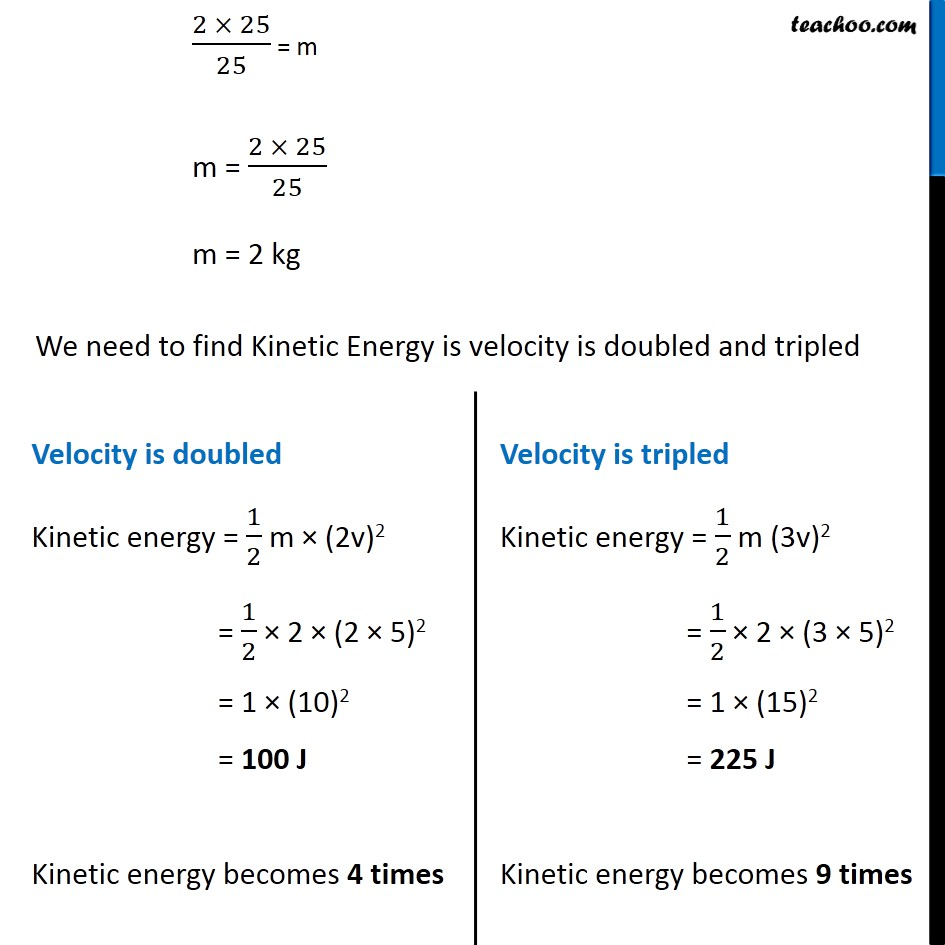Questions from Inside the chapter

Class 9
Chapter 11 Class 9 - Work and EnergyLearn in your speed, with individual attention - Teachoo Maths 1-on-1 Class

### Transcript

Question 3 Page 152 The kinetic energy of an object of mass, m moving with a velocity of 5 m s–1 is 25 J. What will be its kinetic energy when its velocity is doubled? What will be its kinetic energy when its velocity is increased three times? Mass of the object = m Velocity of the object = v = 5 m/s Kinetic energy = 25 J Kinetic energy = 1/2 mv2 25 = 1/2 × m × 52 25 = 1/2 × m × 25 (2 × 25)/25 = m m = (2 × 25)/25 m = 2 kg We need to find Kinetic Energy is velocity is doubled and tripled Velocity is doubled Kinetic energy = 1/2 m × (2v)2 = 1/2 × 2 × (2 × 5)2 = 1 × (10)2 = 100 J Kinetic energy becomes 4 times Velocity is tripled Kinetic energy = 1/2 m (3v)2 = 1/2 × 2 × (3 × 5)2 = 1 × (15)2 = 225 J Kinetic energy becomes 9 times